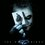# Very basic geometry theorems.Try to find it's difficult applications, and prove them

Right Angles- All right angles are congruent.

Straight Angles- All straight angles are congruent.

Congruent Supplements- Supplements of the same angle, or congruent angles, are congruent.
Congruent Complements- Complements of the same angle, or congruent angles, are congruent.
Linear Pair- If two angles form a linear pair, they are supplementary.

Vertical Angles- Vertical angles are congruent.

Triangle Sum- The sum of the interior angles of a triangle is 180º.

Exterior Angle- The measure of an exterior angle of a triangle is equal to the sum of the measures of the two non-adjacent interior angles.

The measure of an exterior angle of a triangle is greater than either non-adjacent interior angle.

Base Angle Theorem-

(Isosceles Triangle) If two sides of a triangle are congruent, the angles opposite these sides are congruent.

Base Angle Converse-

(Isosceles Triangle) If two angles of a triangle are congruent, the sides opposite these angles are congruent.Note by Sattik Biswas
5 years, 4 months ago

This discussion board is a place to discuss our Daily Challenges and the math and science related to those challenges. Explanations are more than just a solution — they should explain the steps and thinking strategies that you used to obtain the solution. Comments should further the discussion of math and science.

When posting on Brilliant:

• Use the emojis to react to an explanation, whether you're congratulating a job well done , or just really confused .
• Ask specific questions about the challenge or the steps in somebody's explanation. Well-posed questions can add a lot to the discussion, but posting "I don't understand!" doesn't help anyone.
• Try to contribute something new to the discussion, whether it is an extension, generalization or other idea related to the challenge.

MarkdownAppears as
*italics* or _italics_ italics
**bold** or __bold__ bold
- bulleted- list
• bulleted
• list
1. numbered2. list
1. numbered
2. list
Note: you must add a full line of space before and after lists for them to show up correctly
paragraph 1paragraph 2

paragraph 1

paragraph 2

[example link](https://brilliant.org)example link
> This is a quote
This is a quote
    # I indented these lines
# 4 spaces, and now they show
# up as a code block.

print "hello world"
# I indented these lines
# 4 spaces, and now they show
# up as a code block.

print "hello world"
MathAppears as
Remember to wrap math in $$ ... $$ or $ ... $ to ensure proper formatting.
2 \times 3 $2 \times 3$
2^{34} $2^{34}$
a_{i-1} $a_{i-1}$
\frac{2}{3} $\frac{2}{3}$
\sqrt{2} $\sqrt{2}$
\sum_{i=1}^3 $\sum_{i=1}^3$
\sin \theta $\sin \theta$
\boxed{123} $\boxed{123}$

Sort by:

So you want us to derive the entirety of Euclidean geometry again? :P

- 5 years, 4 months ago

No. I mean if you want to do fine go ahead. What I am trying to say is that this are among some of the very basic theorems, using these basic theorems many difficult theorems has been proved. So I am just telling to explore that world a little bit.

- 5 years, 4 months ago

Oh okay, I didn't mean for you to get offended or anything.
Perhaps this belongs more in a wiki?

- 5 years, 4 months ago

Yes it does sir.

- 5 years, 4 months ago